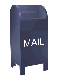# Factor Models

Classical Markowitz portfolio theory uses the historical returns of a portfolio of stocks to build a covariance matrix. The covariance matrix can then be used to estimate the global minimum variance portfolio and the tangency portfolio.

This is a predictive model. The implication is that at time t which directly follows the historical data used to build the covariance, the portfolio return will be $μ$ and the variance will be $σ2$. The return and the variance are entirely dependent on the time series for the portfolio components.

The popular portfolio optimization software (MSCI/BARRA, Axioma and Sungard's APT) all use some form of factor model to construct the covariance matrix used for portfolio optimization. The reasons to use factor models to construct the covariance matrix include:

1. Computational cost

For a large universe of assets (thousands of stocks, for example) constructing a covariance matrix is computationally expensive. Factor models greatly reduce the amount of computation that must be performed.

2. Estimation Error

The covariance matrix is calculated using the time series means. The error in the estimation of the mean is high, so the error in the estimation of the covariance is high as well. The efficient frontier actually exists within a wide band of error.

Factor models reduce the estimation error in the covariance. I hope to perform some experiments to demonstrate this.

3. The Covariance Matrix Cannot be Properly Estimated

In many cases the universe of possible assets for the portfolio is large (for example, the thousand stocks of the Russell 1000). However, the sample size that is used to estimate the covariance matrix is smaller than the number of assets. Estimating the covariance matrix from the sample set may result in a covariance matrix that cannot be inverted. Or if it can be inverted the error will be very high. Here again a factor model avoids this problem.

There are three types of factor model:

1. Macroeconomic
2. Statistical
3. Fundamental

Statistical and fundamental factor models are used to build asset portfolios for investment.

Statistical factor models use Principle Component Analysis (PCA) to decompose returns into a set of statistical factors. This has the attraction of simplicity. The PCA algorithm finds the constituent factors. The draw back is that the actual nature of these factors is unknown and some of the factors may be the result of noise.

The portfolio construction tool maker Axioma takes an interesting approach to this problem (Axioma Insight US Q1 2012). They look at the correlation between the first five statistical factors (the eigen vectors) and a variety of fundamental factors. They examine the confidence interval and those factors with a correlation greater than 0.2 and a confidence interval of 90% are considered valid. Even with the confidence interval measurement it seems possible that this could be a random correlation.

A fundamental factor model attempts to define the factors that explain asset returns. In the ideal case the factor model completely describes the factors contributing to the portfolio return (and hence, variance).

This begs the question... What are these fundamental factors? Here are some examples:

 Size (market capitalization) Industry membership Volatility CVaR (a better risk measure than volatility) Beta with the benchmark index Medium term momentum (cumulative return over 250 days, excluding the most recent 20 day period). Short term momentum Value Growth Stock specific factors Interest rate Liquidity Leverage

Not all assets (stocks) are equally effected by all factors. Since there are many factors that could influence a stock's return it is difficult to know which factors to include and which factors to exclude.

Exposure of a stock to many of these factors changes very slowly. For example, the exposure of a stock to the "Value" factor will change very slowly, if at all.

#### Fundamental Factor Model Examples